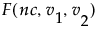To compute power, you make use of the noncentral F distribution. The formula (O’Brien and Lohr 1984) is given as follows:
Power = Prob(F > Fcrit, ν1, ν2, nc)
 • F is distributed as the noncentralandis the 1 - α quantile of the F distribution with ν1 and ν2 degrees of freedom.
 • ν1 = r -1 is the numerator df.
 • ν2 = r(n -1) is the denominator df.
 • n is the number per group.
 • r is the number of groups.
 • nc = n(CSS)/σ2 is the non-centrality parameter.
 • μg is the mean of the gth group.
 • μ is the overall mean.
 • σ2 is estimated by the mean squared error (MSE).

Help created on 9/19/2017